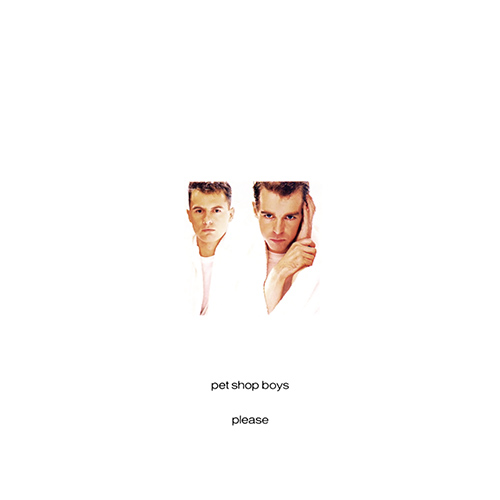## Albums | Songs | Videos | Lyrics

### Singles### Two Divided By Zero

(Two divided by zero, zero)
(Two divided by zero, zero, zero)

Let's not go home
We'll catch the late train
I've got enough money
To pay on the way
When the postman calls
He'll deliver the letter
I've explained everything
It's better that way

(Divided by, divided by)
I think they heard a rumour
(Divided by, divided by)
Or someone tipped them off
(Divided by, divided by)
It's better to go sooner
(Divided by, divided by)
Than call it all off

We'll catch a plane to New York
And a cab going down
Cross the bridges and tunnels
Straight into town
Tomorrow morning
We'll be miles away
On another continent
And another day

(Divided by, divided by)
Let's not go home
(Divided by, divided by)
Or call it a day
(Divided by, divided by)
You won't be alone
(Divided by, divided by)
Let's run away

(Two divided by zero, zero)
(Two divided by zero, zero, zero)
(Zero)
(Divided by, divided by)
(Divided by, divided by zero, zero)
(Divided by, divided by)
Better to go sooner
(Divided by, divided by)
Let's run away

So why hang around
For the deed to be done?
You can give it all up
For a place in the sun
When the postman calls
We'll be miles away
On a plane to New York
And another day

(Divided by, divided by)
I think they heard a rumour
(Divided by, divided by)
Or someone tipped them off
(Divided by, divided by)
Better to go sooner
(Divided by, divided by)
Than call it all off

(Divided by, divided by)